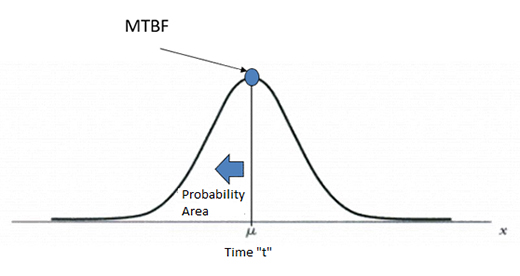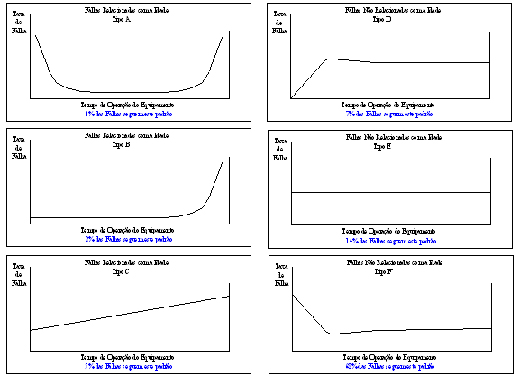#Quality Digest

Featured Product
This Week in Quality Digest Live
Operations Features
Huw Thomas
A long-awaited expansion of workers’ rights
Tom Rish
Keep it organized and ready for inspection at any time
ISO
MSMEs are encouraged to uphold the highest standards
engineering.com
It’s actually the differences in a twin that are most useful
Del Williams
Preventing damage caused by large, suspended particles
Operations News
Maximum work envelope in a small footprint
On-demand pipe flow measurement, no process interruptions
Extends focus on data-driven explainability and adds customizability
For workshop or lab environments
New capability delivers deeper productivity insights to help manufacturers meet labor challenges
Runs on NTT’s Docomo network
Day and a half workshop to learn, retain, and transfer GD&T knowledge across an organization
Making designs a physical reality with the know-how to make more
Major ERP projects take six months longer than companies were toldBio

Operations

## What Does MTBF Mean?

### Four points of consideration

Published: Tuesday, March 19, 2019 - 12:02

Within maintenance management, the term MTBF (mean time between failures) is the most important key performance indicator after physical availability.

Unlike MTTF (mean time to failure), which relates directly to available equipment time, MTBF also adds up the time spent inside a repair. That is, it starts its count from a certain failure and only stops when the fault is remedied, and the equipment restarted and performing again. According to ISO 12489, this indicator can only be used for repairable equipment, and MTTF is the equivalent of nonrepairable equipment.

The graphic below illustrates these occurrences:Figure 1: Mean time between failures

To calculate the MTBF in figure 1, we add the times T1 and T2 and divide by two. That is, the average of all times between one failure and another, as well as its return, is calculated. It is, therefore, a simple mathematical calculation. But what does MTBF mean?

Generally speaking, this indicator is associated with a reliability quality of assets or asset systems, and may even reach a repairable item, although it is rare to have data available to that detail. Maintenance managers set some benchmark numbers and track performance on a chart over time. In general, the higher the MTBF, the better; or the fewer times of breaks and repairs over the analyzed period.

Once we have determined the concepts, some particularities need to be answered:
1. Can we establish periodicity of a maintenance plan based on MTBF time?
2. Can we calculate failure rates based on MTBF?
3. Can we calculate probability of failure based on MTBF?
4. If the MTBF of an asset or system is 200 hours, after that time will it fail?

Let’s answer each of these questions separately.

### 1. Can we establish periodicity of a maintenance plan based on MTBF time?

The MTBF is an average number calculated from a set of values. That is, these values can be grouped into a histogram to generate a data distribution where the average value is its MTBF, or the average of the data. Imagine that this distribution follows the Gaussian law, and we have a normal curve that was modeled based on the failure data. The chart below shows that the MTBF is positioned in the middle of the chart.Figure 2: Normal distribution model

In a modeled PDF (probability density of failure) curve, the mean value, or MTBF, will occur after 50 percent of the failure frequencies have occurred. If we implement the preventive plan with a frequency equal to the MTBF time, it will already have a 50-percent probability of failing. Therefore, the MTBF is not a number that indicates the optimal time for a scheduled intervention.

### 2. Can we calculate failure rates based on MTBF?

Considering the modeling of the failure data to calculate the MTBF, it’s only possible in the exponential distribution to fix a value where the failure rate is the inverse of the MTBF:

MTBF = 1 / ʎ

In this distribution, the MTBF time already corresponds to 63.2 percent probability of failure.Figure 3: Exponential distribution model

With any modeling other than exponential, the failure rate will be variable and time-dependent, so its calculation will also depend on factors such as the probability density function f(t) and the reliability function R(t):

ʎ(t) = h(t) = f(t) / R(t)

Although the exponential distribution is the most adopted in reliability projects, which would generate a constant failure rate over time, most of the assets have variations within their “bathtub curve,” as exemplified by Moubray:Figure 4: Different bathtub curves (Moubray, 1997)

This means that the exponential expression is not best suited to reflect the behavior of most assets in an industrial plant.

### 3. Can we calculate probability of failure based on MTBF?

As seen above, only in the exponential distribution is there a constant failure rate that can be calculated as the inverse of the MTBF. In this case, yes, we can calculate the probability of failure of an asset using the formula below:

f(t) = ʎˑexp(-ʎt)

For other models where the failure rate depends on the time, it is only possible to calculate the probability of failure through data modeling and determination of a parametric statistical curve.

### 4. If the MTBF of an asset or system is 200 hours, after that time will it fail?

What exactly does that number mean? It was shown that MTBF isn’t used as a maintenance plan frequency. According to the concepts explained above, this time means nothing because it is not comparable to its history over months. If the parametric model governing the behavior of the assets in a reliability study is not determined, the time of 200 hours has no meaning for a probability of failure. In the case of an MTBF provided by equipment manufacturers that is different, through life tests it’s possible to determine exponential curves and thus calculate the time in which there will be 63.2 percent of sample failures.

I hope this discussion has helped us to reflect on the definitions of an indicator that is both used and also so misunderstood within industrial maintenance management.

### Discuss### Teofilo Cortizo

With a master’s degree in industrial engineering, Teofilo Cortizo is a reliability engineer with more than a decade of experience in the corporate world.

### ISO 12849

Hi,

I've been on the ISO website at https://www.iso.org/obp/ui/#home - went looking for ISO 12849 but can't seem to find it - any chance of posting a link?

Thanks

### ISO 12489

Hi Ronnie That was a typo in the article. It should have read ISO 12489 https://www.iso.org/standard/51456.html

### Found it - thanks! Also

Found it - thanks! Also thanks for a good article!